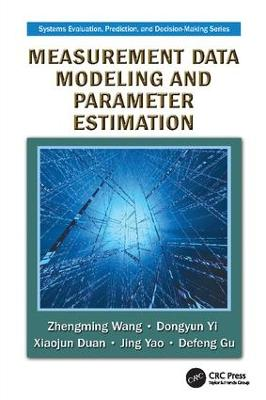•# Measurement Data Modeling and Parameter Estimation - Systems Evaluation, Prediction, and Decision-Making (Paperback)

(author), (author), (author), (author), (author)
£61.99
Paperback 553 Pages / Published: 31/05/2017
• We can order this

Usually dispatched within 3 weeks

Measurement Data Modeling and Parameter Estimation integrates mathematical theory with engineering practice in the field of measurement data processing. Presenting the first-hand insights and experiences of the authors and their research group, it summarizes cutting-edge research to facilitate the application of mathematical theory in measurement and control engineering, particularly for those interested in aeronautics, astronautics, instrumentation, and economics.

Requiring a basic knowledge of linear algebra, computing, and probability and statistics, the book illustrates key lessons with tables, examples, and exercises. It emphasizes the mathematical processing methods of measurement data and avoids the derivation procedures of specific formulas to help readers grasp key points quickly and easily. Employing the theories and methods of parameter estimation as the fundamental analysis tool, this reference:

Introduces the basic concepts of measurements and errorsApplies ideas from mathematical branches, such as numerical analysis and statistics, to the modeling and processing of measurement dataExamines methods of regression analysis that are closely related to the mathematical processing of dynamic measurement dataCovers Kalman filtering with colored noises and its applications

Converting time series models into problems of parameter estimation, the authors discuss modeling methods for the true signals to be estimated as well as systematic errors. They provide comprehensive coverage that includes model establishment, parameter estimation, abnormal data detection, hypothesis tests, systematic errors, trajectory parameters, and modeling of radar measurement data. Although the book is based on the authors' research and teaching experience in aeronautics and astronautics data processing, the theories and methods introduced are applicable to processing dynamic measurement data across a wide range of fields.

Publisher: Taylor & Francis Ltd
ISBN: 9781138114920
Number of pages: 553
Weight: 1039 g
Dimensions: 235 x 156 mm